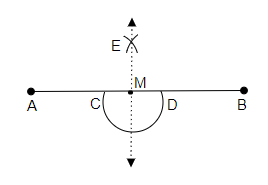# Draw any line segment $\overline{\mathrm{AB}}$. Mark any point $\mathrm{M}$ on it. Through $\mathrm{M}$, draw a perpendicular to $\overline{\mathrm{AB}}$. (use ruler and compasses)

To do:

We have to draw a perpendicular to $\overline{AB}$.

Solution:Steps of construction:

(i) Let us draw a line segment $\overline{AB}$ and mark a point M on it.

(ii) Now, by taking M as a centre let's draw an arc intersecting the line segment $\overline{AB}$ and name the intersecting points as C and D respectively.

(iii) Now, by pointing the pointer of the compasses at C as the centre with a measure of radius greater than C to M let's draw an arc.

(iv) Similarly, by pointing the pointer at D as the centre with the same measure let's draw another arc. let these two arcs intersect each other at point E.

(v) Then, join the points M and E.

(vi) Therefore, $\overline{EM}$ is perpendicular to $\overline{AB}$.

Updated on: 10-Oct-2022

42 Views